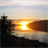# QlikView App Dev

Discussion Board for collaboration related to QlikView App Development.

Announcements
Customer & Partners, DEC. 9, 11 AM ET: Qlik Product & Strategy Roadmap Session: Data Analytics REGISTER NOW
cancel
Showing results for
Did you mean:Not applicable

## Query Help

Hi just wondering if anyone can help with a query.

In the attached excel file you can see my calculations and below is explaining what I am trying to do, if i am not clear i will try make it clearer.

As you can see each ID has multiple different incomes. What I want to calculate is too different things I am just not sure if my query is correct.

There are two different calculations I want to make when the sum of sales is in the range of 0 to 15 and when the sum of sales is between 15 and 25.

When an account has gross sales between 0 - 15 the calculation you get Fee + sum of Commission so for ID 1111 this would be 5+ 25 = 30

When an account has gross sales between 15 and 25 It is calculated as Charge - sum of sales + sum of commission amount. So for ID 2222 it would be 15-7 = 8 + 6 = 14.

Then ID 3333 the gross of sales is  above 25 so I don't need to calculate it.

I have created a straight table with the Dimension of ID and three expressions.

Label sales and it's sum(Sales)

Commission Due as the label and the query which I am unsure about this is what I have attempted but I don't seem to be getting back the results I am looking for.

If(Sum(Sales)>15 and Sum(Sales)<25 = Charge-(Sum(Sales))+Sum(Commission), If (Sum(Sales)<15 = Sum(Commission))+[Fees])

Then label Charge and value Charge.

1 Solution

Accepted SolutionsSpecialist

Hi

Aggr(if(sum(Sales) <= 15,sum(Commission) + sum(Fees),if(sum(Sales)>15,Charge-(Sum(Sales))+Sum(Commission),0)),ID)

5 RepliesSpecialist

Hi

Aggr(if(sum(Sales) <= 15,sum(Commission) + sum(Fees),if(sum(Sales)>15,Charge-(Sum(Sales))+Sum(Commission),0)),ID)Specialist

use Only Aggr function is have onle value for the Charge field

Aggr(if(sum(Sales) <= 15,sum(Commission) + sum(Fees),if(sum(Sales)>15,only(Charge)- (Sum(Sales))+Sum(Commission),0)),ID)Not applicable
AuthorMaster III

If not, please make clear with what part of this topic we can still help you.